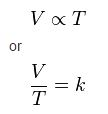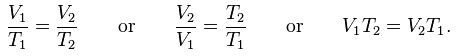# Charles law

The principle that the volume of a given mass of an ideal gas is proportional to its temperature as long as its pressure remains constant. Charles's law is a subcase of the ideal gas law. Charles' law (also known as the law of volumes) is an experimental gas law that describes how gases tend to expand when heated. A modern statement of Charles' law is: When the pressure on a sample of a dry gas is held constant, the Kelvin temperature and the volume will be directly related.this directly proportional relationship can be written as:#### where:

V is the volume of the gas

T is the temperature of the gas (measured in Kelvin).

k is a constant

This law describes how a gas expands as the temperature increases; conversely, a decrease in temperature will lead to a decrease in volume. For comparing the same substance under two different sets of conditions, the law can be written as:The equation shows that, as absolute temperature increases, the volume of the gas also increases in proportion.